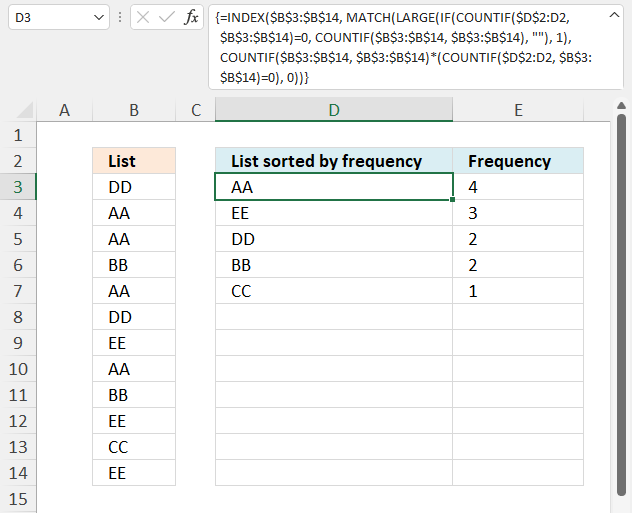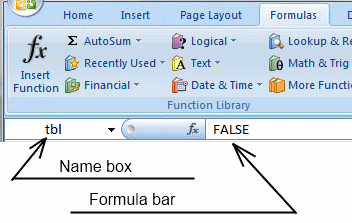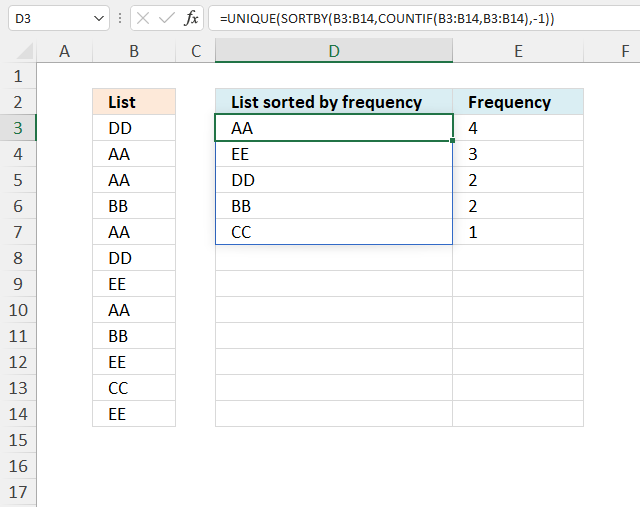Author: Oscar Cronquist Article last updated on November 10, 2021This article demonstrates a formula that sorts cell values by their frequency, in other words, how many times a value is repeated in the list.

1. Sort column based on the frequencyQuestion:

How do I create a new unique distinct list from a column? I also want the list sorted from large to small by the number of times a value is repeated?

Array formula in cell D3:

=INDEX(\$B\$3:\$B\$14, MATCH(LARGE(IF(COUNTIF(\$D\$2:D2, \$B\$3:\$B\$14)=0, COUNTIF(\$B\$3:\$B\$14, \$B\$3:\$B\$14), ""), 1), COUNTIF(\$B\$3:\$B\$14, \$B\$3:\$B\$14)*(COUNTIF(\$D\$2:D2, \$B\$3:\$B\$14)=0), 0))

1.1 How to create an array formula

1. Copy (Ctrl + c) and paste (Ctrl + v) array formula into formula bar. See the picture below.
2. Press and hold Ctrl + Shift.
3. Press Enter once.
4. Release all keys.Recommended article

A beginners guide to Excel array formulas

Array formulas allows you to do advanced calculations not possible with regular formulas.

How to copy array formula in cell D3

1. Select cell D3
2. Copy cell (Ctrl + c)
3. Select cell range D4:D8
4. Paste (Ctrl + v)

1.2 Explaining array formula in cell D3

Step 1 - Count previous items

The COUNTIF function calculates the number of cells that is equal to a condition.

COUNTIF(rangecriteria)

COUNTIF(\$D\$2:D2, B3:B14)

becomes

COUNTIF("List sorted by frequency", {"DD";"AA";"AA";"BB";"AA";"DD";"EE";"AA";"BB";"EE";"CC";"EE"})

and returns

{0; 0; 0; 0; 0; 0; 0; 0; 0; 0; 0; 0}.

Step 2 - Identify positions of previous items

The equal sign compares each value in the array to 0 (zero), the result is a boolean value TRUE or FALSE.

COUNTIF(\$D\$2:D2, B3:B14)=0

becomes

{0; 0; 0; 0; 0; 0; 0; 0; 0; 0; 0; 0}=0

and returns

{TRUE; TRUE; TRUE; TRUE; TRUE; TRUE; TRUE; TRUE; TRUE; TRUE; TRUE; TRUE}.

Step 3 - Count items in the list

COUNTIF(B3:B14, B3:B14)

becomes

COUNTIF({"DD"; "AA"; "AA"; "BB"; "AA"; "DD"; "EE"; "AA"; "BB"; "EE"; "CC"; "EE"}, {"DD"; "AA"; "AA"; "BB"; "AA"; "DD"; "EE"; "AA"; "BB"; "EE"; "CC"; "EE"})

and returns

{2; 4; 4; 2; 4; 2; 3; 4; 2; 3; 1; 3}.

Step 4 - Create array containing count for new values

The IF function returns one value if the logical test is TRUE and another value if the logical test is FALSE.

IF(logical_test, [value_if_true], [value_if_false])

IF(COUNTIF(\$D\$2:D2, B3:B14)=0, COUNTIF(B3:B14, B3:B14), "")

becomes

IF({TRUE; TRUE; TRUE; TRUE; TRUE; TRUE; TRUE; TRUE; TRUE; TRUE; TRUE; TRUE}, {2; 4; 4; 2; 4; 2; 3; 4; 2; 3; 1; 3}, "")

and returns

{2; 4; 4; 2; 4; 2; 3; 4; 2; 3; 1; 3}.

Step 5 - Get largest count

The LARGE function calculates the k-th largest value from an array of numbers.

LARGE(arrayk)

LARGE(IF(COUNTIF(\$D\$2:D2, B3:B14)=0, COUNTIF(B3:B14, B3:B14), ""), 1)

becomes

LARGE({2; 4; 4; 2; 4; 2; 3; 4; 2; 3; 1; 3}, 1)

and returns 4.

Step 5 - Find the position in the array of the largest count

The MATCH function returns the relative position of an item in an array or cell reference that matches a specified value in a specific order.

MATCH(lookup_valuelookup_array, [match_type])

MATCH(LARGE(IF(COUNTIF(\$D\$2:D2, B3:B14)=0, COUNTIF(B3:B14, B3:B14), ""), 1), COUNTIF(B3:B14, B3:B14)*(COUNTIF(\$D\$2:D2, B3:B14)=0), 0)

becomes

MATCH(4, COUNTIF(B3:B14, B3:B14)*(COUNTIF(\$D\$2:D2, B3:B14)=0), 0)

becomes

MATCH(4, {2; 4; 4; 2; 4; 2; 3; 4; 2; 3; 1; 3}), 0)

and returns 2.

Step 6 - Get value based on position

The INDEX function returns a value from a cell range, you specify which value based on a row and column number.

INDEX(array[row_num][column_num], [area_num])

INDEX(B3:B14, MATCH(LARGE(IF(COUNTIF(\$D\$2:D2, B3:B14)=0, COUNTIF(B3:B14, B3:B14), ""), 1), COUNTIF(B3:B14, B3:B14)*(COUNTIF(\$D\$2:D2, B3:B14)=0), 0))

becomes

INDEX(B3:B14, 2)

becomes

INDEX({"DD";"AA";"AA";"BB";"AA";"DD"; "EE";"VV";"BB";"EE";"VV";"EE"}, 2)

and returns "AA" in cell D3.The following formula returns the count of the corresponding value in column D.

Formula in E3:

=COUNTIF(\$B\$3:\$B\$14, D3)

How to use the COUNTIF function

Counts the number of cells that meet a specific condition.

2. Sort column by frequency - Excel 365Formula in cell D3:

=UNIQUE(SORTBY(B3:B14,COUNTIF(B3:B14,B3:B14),-1))

2.1 Explaining formula in cell D3

Step 1 - Count each value

The COUNTIF function calculates the number of cells that is equal to a condition.

COUNTIF(rangecriteria)

COUNTIF(B3:B14, B3:B14)

becomes

COUNTIF({"DD"; "AA"; "AA"; "BB"; "AA"; "DD"; "EE"; "AA"; "BB"; "EE"; "CC"; "EE"}, {"DD"; "AA"; "AA"; "BB"; "AA"; "DD"; "EE"; "AA"; "BB"; "EE"; "CC"; "EE"})

and returns

{2; 4; 4; 2; 4; 2; 3; 4; 2; 3; 1; 3}.

Step 2 - Sort values by count

The SORTBY function allows you to sort values from a cell range or array based on a corresponding cell range or array. It sorts values by column but keeps rows.

SORTBY(array, by_array1, [sort_order1], [by_array2, sort_order2], …)

SORTBY(B3:B14, COUNTIF(B3:B14, B3:B14), -1)

becomes

SORTBY(B3:B14, {2; 4; 4; 2; 4; 2; 3; 4; 2; 3; 1; 3}, -1)

becomes

SORTBY({"DD"; "AA"; "AA"; "BB"; "AA"; "DD"; "EE"; "AA"; "BB"; "EE"; "CC"; "EE"}, {2; 4; 4; 2; 4; 2; 3; 4; 2; 3; 1; 3}, -1)

and returns

{"AA"; "AA"; "AA"; "AA"; "EE"; "EE"; "EE"; "DD"; "BB"; "DD"; "BB"; "CC"}.

Step 3 - Filter unique distinct values

The UNIQUE function extracts unique distinct rows from the array.

UNIQUE(array,[by_col],[exactly_once])

UNIQUE(SORTBY(B3:B14, COUNTIF(B3:B14, B3:B14), -1))

becomes

UNIQUE({"AA"; "AA"; "AA"; "AA"; "EE"; "EE"; "EE"; "DD"; "BB"; "DD"; "BB"; "CC"})

and returns {"AA"; "EE"; "DD"; "BB"; "CC"}.